Related Articles

# Stacking in Machine Learning

• Last Updated : 02 Sep, 2020

Stacking:
Stacking is a way of ensembling classification or regression models it consists of two-layer estimators. The first layer consists of all the baseline models that are used to predict the outputs on the test datasets. The second layer consists of Meta-Classifier or Regressor which takes all the predictions of baseline models as an input and generate new predictions.

Stacking Architecture: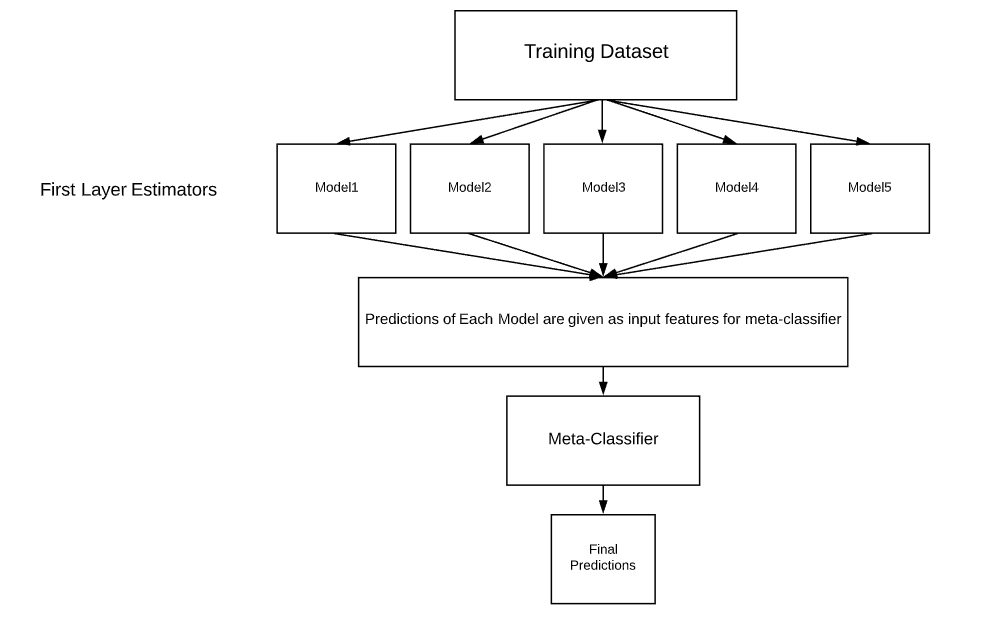Stacking Architecture

mlxtend:
Mlxtend (machine learning extensions) is a Python library of useful tools for day-to-day data science tasks. It consists of lots of tools that are useful for data science and machine learning tasks for example:

1. Feature Selection
2. Feature Extraction
3. Visualization
4. Ensembling

and many more.

This article explains how to implement Stacking Classifier on the classification dataset.

Why Stacking?
Most of the Machine-Learning and Data science competitions are won by using Stacked models. They can improve the existing accuracy that is shown by individual models. We can get most of the Stacked models by choosing diverse algorithms in the first layer of architecture as different algorithms capture different trends in training data by combining both of the models can give better and accurate results.

Installation of libraries on the system:

pip install mlxtend
pip install pandas
pip install -U scikit-learn

Code: Import Required Libraries:

 `import` `pandas as pd``import` `matplotlib.pyplot as plt``from` `mlxtend.plotting ``import` `plot_confusion_matrix``from` `mlxtend.classifier ``import` `StackingClassifier``from` `sklearn.model_selection ``import` `train_test_split``from` `sklearn.preprocessing ``import` `StandardScaler``from` `sklearn.linear_model ``import` `LogisticRegression``from` `sklearn.neighbors ``import` `KNeighborsClassifier``from` `sklearn.naive_bayes ``import` `GaussianNB ``from` `sklearn.metrics ``import` `confusion_matrix``from` `sklearn.metrics ``import` `accuracy_score`

 `df ``=` `pd.read_csv(``'heart.csv'``)    ``# loading the dataset``df.head()                        ``# viewing top 5 rows of dataset`

Output: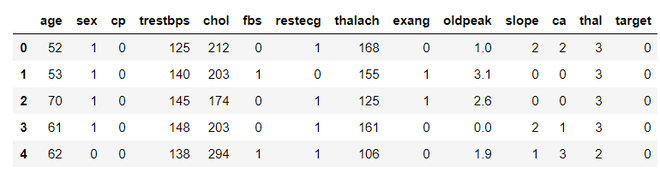Code:
 `# Creating X and y for training``X ``=` `df.drop(``'target'``, axis ``=` `1``)``y ``=` `df[``'target'``]`

Code: Splitting Data into Train and Test

 `# 20 % training dataset is considered for testing``X_train, X_test, y_train, y_test ``=` `train_test_split(X, y, test_size ``=` `0.2``, random_state ``=` `42``)`

Code: Standardising Data

 `# initializing sc object``sc ``=` `StandardScaler()  ``# variables that needed to be transformed``var_transform ``=` `[``'thalach'``, ``'age'``, ``'trestbps'``, ``'oldpeak'``, ``'chol'``]``X_train[var_transform] ``=` `sc.fit_transform(X_train[var_transform])   ``# standardising training data ``X_test[var_transform] ``=` `sc.transform(X_test[var_transform])            ``# standardising test data``print``(X_train.head())`

Output: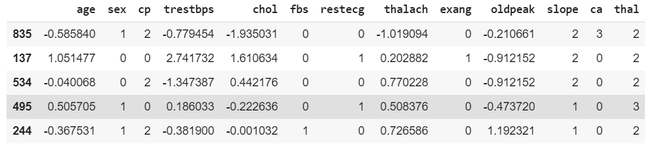Code: Building First Layer Estimators
 `KNC ``=` `KNeighborsClassifier()   ``# initialising KNeighbors Classifier``NB ``=` `GaussianNB()              ``# initialising Naive Bayes`

Let’s Train and evaluate with our first layer estimators to observe the difference in the performance of the stacked model and general model

Code: Training KNeighborsClassifier

 `model_kNeighborsClassifier ``=` `KNC.fit(X_train, y_train)   ``# fitting Training Set``pred_knc ``=` `model_kNeighborsClassifier.predict(X_test)   ``# Predicting on test dataset`

Code: Evaluation of KNeighborsClassifier

 `acc_knc ``=` `accuracy_score(y_test, pred_knc)  ``# evaluating accuracy score``print``(``'accuracy score of KNeighbors Classifier is:'``, acc_knc ``*` `100``)`

Output: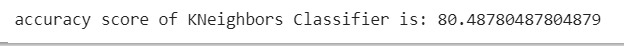Code: Training Naive Bayes Classifier
 `model_NaiveBayes ``=` `NB.fit(X_train, y_train)``pred_nb ``=` `model_NaiveBayes.predict(X_test)`

Code: Evaluation of Naive Bayes Classifier

 `acc_nb ``=` `accuracy_score(y_test, pred_nb)``print``(``'Accuracy of Naive Bayes Classifier:'``, acc_nb ``*` `100``)`

Output: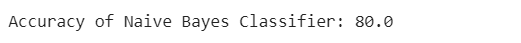Code: Implementing Stacking Classifier
 `lr ``=` `LogisticRegression()  ``# defining meta-classifier``clf_stack ``=` `StackingClassifier(classifiers ``=``[KNC, NB], meta_classifier ``=` `lr, use_probas ``=` `True``, use_features_in_secondary ``=` `True``)`
• `use_probas=True` indicates the Stacking Classifier uses the prediction probabilities as an input instead of using predictions classes.
• `use_features_in_secondary=True` indicates Stacking Classifier not only take predictions as an input but also uses features in the dataset to predict on new data.

Code: Training Stacking Classifier

 `model_stack ``=` `clf_stack.fit(X_train, y_train)   ``# training of stacked model``pred_stack ``=` `model_stack.predict(X_test)       ``# predictions on test data using stacked model`

Code: Evaluating Stacking Classifier

 `acc_stack ``=` `accuracy_score(y_test, pred_stack)  ``# evaluating accuracy``print``(``'accuray score of Stacked model:'``, acc_stack ``*` `100``)`

Output: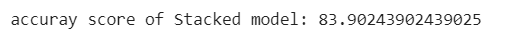Our both individual models scores an accuracy of nearly 80% and our Stacked model got an accuracy of nearly 84%.By Combining two individual models we got a significant performance improvement.

Code:

 `model_stack ``=` `clf_stack.fit(X_train, y_train)   ``# training of stacked model``pred_stack ``=` `model_stack.predict(X_test)       ``# predictions on test data using stacked model`

Code: Evaluating Stacking Classifier

 `acc_stack ``=` `accuracy_score(y_test, pred_stack)  ``# evaluating accuracy``print``(``'accuray score of Stacked model:'``, acc_stack ``*` `100``)`

Output:Our both individual models scores an accuracy of nearly 80% and our Stacked model got an accuracy of nearly 84%. By Combining two individual models we got a significant performance improvement.

Attention reader! Don’t stop learning now. Get hold of all the important Machine Learning Concepts with the Machine Learning Foundation Course at a student-friendly price and become industry ready.

My Personal Notes arrow_drop_up